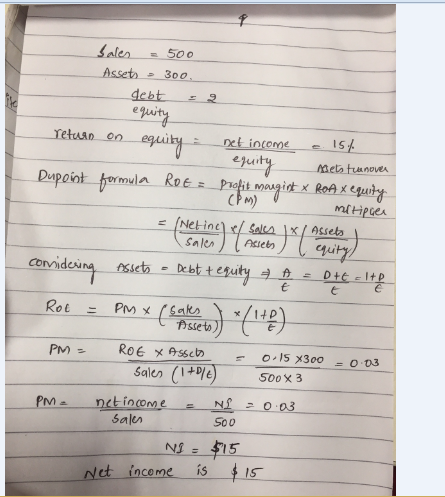# Question & Answer: A firm has sales of \$500 total assets of \$300 and a debt/equity ratio of 2.00. If it returns on…..

A firm has sales of \$500 total assets of \$300 and a debt/equity ratio of 2.00. If it returns on equity is 15 percent what is its net income?